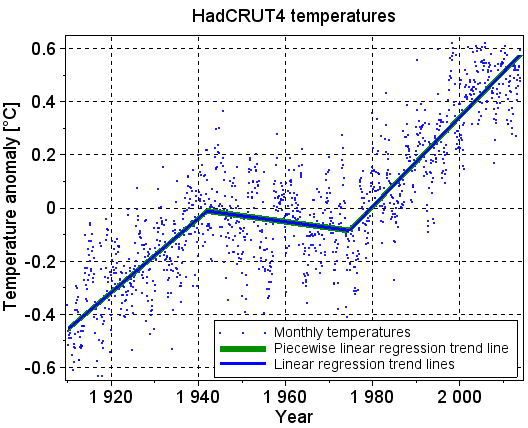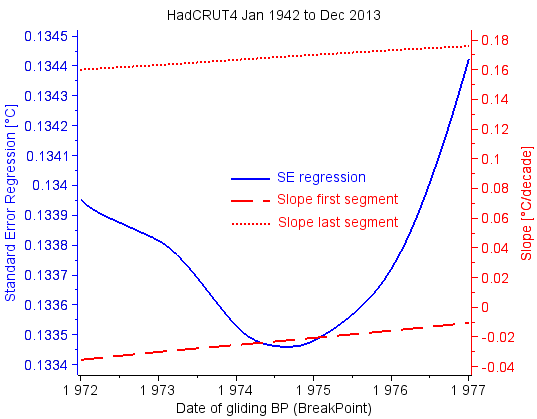## søndag 22. juni 2014

### Piecewise linear regression applied to temperature trends

This is the fifth blog post in a series of five that analyse trends in the global surface temperatures. The posts put emphasis on the mathematics and the statistics used in the analyses. The posts are numbered 1 to 5. They should be read consecutively.

Post 1    Linear regression analysis
Post 2    Hypothesis testing of temperature trends
Post 3    Confidence intervals around temperature trend lines
Post 4    Statistical power of temperature trends
Post 5    Piecewise linear regression applied to temperature trends

The posts are gathered in this pdf document.

Start of post 5 Piecewise linear regression applied to temperature trends

The temperature trend line from December 2000 to December 2013 is flat, while the one from January 1984 to November 2000 increases with 0.22°C/decade, as shown with the two blue lines in Figure 5.1.Figure 5.1: Monthly temperatures in the last 30 years with trend lines

This leads many contrarians to argue that the increasing temperature trend before the turn of the millennium is followed by a flat trend, and they often illustrate their claim with the red schematic line in Figure 5.1. The red line is, however, not based on calculations, and it does not match the monthly temperatures that it claims to represent. The two blue lines are calculated with linear regression analysis, and they represent the temperatures in their segments when the segments are evaluated isolated from each other. But the trend lines are not continuous at the breakpoint between November and December 2000, and they therefore do not represent the trend for the whole time period in Figure 5.1.

We may calculate a piecewise linear trend line that is continuous at the breakpoint. This new trend line is a “best fit” to the temperatures in the whole time period, just as the two blue lines are the best fits for their time periods. The new trend line has an increasing trend also after the turn of the millennium, as the green line in Figure 5.1 shows. It is calculated with piecewise linear regression analysis.

The green piecewise linear regression line in Figure 5.1 does not represent the long term temperature trend in the last 30 years well. The time period is split into two periods that both are too short for reliable long term trend calculations, and the linear regression lines that are calculated separately for the two time periods are far from continuous. The green line do, however, show how misleading the red trend line is.

The best way to represent the long term temperature trend in the last 30 years is to calculate the trend line based on all temperatures in the period, as shown with the trend line in Figure 3.1 in post 3.

Piecewise linear regression analysis should only be used when there is a good reason to assume that there is a change in the trend at the breakpoint(s).

Some claim that there are changes in the long term temperature trend at the end of 1941 and in 1974. Figure 5.2 supports that claim. The blue lines show the trend lines when they are calculated separately in the three segments. They are all statistically significant at the 0.05 significance level, and they are continuous at the breakpoints.Figure 5.2: Monthly temperatures since 1910 with trend lines

Figure 5.2 also shows the green trend line that is calculated with piecewise linear regression analysis with breakpoints at the crossings between the blue lines. The green line is completely covered by the three blue lines, and it is therefore drawn with a broad line to make it visible.

Mathematics

A piecewise linear trend line is shown with the green line in Figure 5.1 and in Figure 5.2. Each yi measurement deviates more or less from the trend line. (1.2) in post 1 defines the deviation (error) ei for the simple case with only one straight trend line. That equation must be extended to handle the more complex piecewise linear trend line. A common way to do this is

b1 is the slope of the first segment, b2 is the change in the slope from segment 1 to segment 2, and bm is the change in the slope from segment (m-1) to segment m. xbp1 is the x value that separates segment 1 and 2, and xbp(m-1) is the x value that separates segment (m-1) and m. u1 is zero when x is less than xbp1 and 1 when greater than xbp1. u(m-1) is zero when x is less than xbp(m-1) and 1 when greater than xbp(m-1).

The Sum of the Squared Errors (SSE) is defined in (1.3) in post 1. In piecewise linear regression analysis we calculate the estimates of a and b1 to bm that minimize SSE, just as we did in the simple case with only one b. For the simple case there is a simple analytical solution to the minimization, as shown in (1.4) and (1.6) in post 1. For piecewise linear regression with many slopes, a curve fitting function may be used to calculate the best estimates of a and b1 to bm. We have used SciLab's datafit() function in the calculations behind the figures.

The breakpoints xbp1 to xbp(m-1) separate the m segments of the piecewise linear regression line. The explanation in the previous paragraphs assumes that the breakpoints are decided in beforehand, i.e. that they are kept at their fixed values when calculating the intercept and the slopes that minimize SSE. The calculation behind Figure 5.2 may be extended to also decide the two breakpoints. This is possible because SSE has well defined minima in the breakpoints. The Standard Error of the Regression (SEregression) is a function of SSE. They have their minima at the same times, as (2.1) in post 2 shows. Figure 5.3 shows that SEregression has a minimum when the breakpoint is in the autumn of 1974. This supports the claim that there is a change in the long term temperature trend in 1974.Figure 5.3: The Standard Error of the Regression has a minimum when the breakpoint of the piecewise linear regression line is in the autumn of 1974.The calculations behind the figure uses the temperatures from 1942 to 2013.

Contrarians often claim that there has been no increase in the global surface temperature in the last 17, 16 or 15 years. We will now check this in more detail.

It is not possible to extend the calculations behind Figure 5.1 to also decide a breakpoint at the turn of the millennium because there is no minimum in SEregression at that time, as shown in Figure 5.4.Figure 5.4: The standard error of the regression has no minimum for a breakpoint at the turn of the millennium. The calculations behind the figure uses the temperatures from 1984 to 2013.

The blue line in Figure 5.4 is almost flat in the start of 1999, but that is not a well defined minimum. We may, however, try to move the breakpoint in Figure 5.1 back in time to the start of 1999, as shown in Figure 5.5.Figure 5.5: Monthly temperatures in the last 30 years with separate regression lines before and after 1999

The intersection between the two blue regression lines is almost between the end of the first line and the start of the last one, but not exactly as in Figure 5.2. The green piecewise linear regression line is almost behind the two blue regression lines, but not completely as in Figure 5.2. The reason for this is that SEregression has well defined minima in the breakpoints in Figure 5.2, but not in the breakpoint in Figure 5.5.

There is no reason to claim that there is a change in the long term temperature trend at the turn of the millennium. But if the contrarians still want to do so, they must put the breakpoint as shown in Figure 5.5. The last segment is only 15 years, which is far too short for evaluating reliable long term climate trends. And the slope in the last segment is definitely positive.

References for the mathematics

Hans von Storch, Francis W. Zwiers. 2001. Statistical Analysis in Climate Research is our main reference for linear regression analysis without breakpoints. See chapter 8.3 'Fitting and Diagnosing Simple Regression Models'.

Derek S. Young. May 2014. PSU course STAT 501 Regression Methods extends the simple model so that the regression line may have many breakpoints. See chapter 19.1 'Piecewise Linear Regression' in Part IV. We have used the formulas for the continuous piecewise regression line.

#### 1 kommentar:

1.Do you think that to dramatically improve your success rate you have to read tons of thick books, buy expensive software and spend countless hours of learning more about Forex?
What I'm going to share with you is something very EASY to use and very POWERFUL at the same time.
Let me give you an EXAMPLE:
Imagine you trade a system that makes 50% winning trades, but another 50% are losing trades. If you increase your odds of winning by only 20%, that would make 70% winning trades and 30% losing trades.
Well, HOW TO accomplish that?
Just pick the best trending pair at the current time and simply follow the trend! I have found ONE INCREDIBLE TOOL that continuously scans the Forex market and picks the most reliable trending pairs for you.
==> http://www.forextrendy.com?ljsjhd8374h
By taking signals in the direction of a strong trend you would REDUCE UNNECESSARY LOSSES and increase the odds of winning. You need to know "how well" the market is trending to avoid very short-term trends.
STOP hunting the market for every potential trade. Pick only the best trending pairs and time frames and DO NOT take any trading signals in the choppy market (unless you know exactly what you are doing).

Successful traders keep it simple and this is the way how the pros made fortunes in the markets - by trading less and making more.
To increase the profitability of any system or robot you are currently using, check out this easy and powerful ultimate solution:
==> http://www.forextrendy.com?ljsjhd8374h